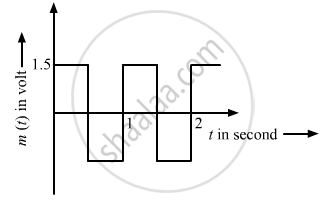# The Carrier Wave of a Signal is Given by C(T) = 3 Sin (8πT) Volt. the Modulating Signal is a Square Wave as Shown. Find Its Modulation Index. - Physics

The carrier wave of a signal is given by C(t) = 3 sin (8πt) volt. The modulating signal is a square wave as shown. Find its modulation index.#### Solution

Modulation index ($\mu$) is the ratio of the amplitude of the modulating signal to the amplitude of the carrier wave.

The generalised equation of a carrier wave is given below:

$c(t) = A_c \sin \omega_c t$
The generalised equation of a modulating wave is given below:
$c_m (t) = A_c \sin \omega_c t + \mu A_c \sin \omega_m t\sin \omega_c t$
Here,
$\mu$  is defined as $\frac{A_m}{A_c}$.
On comparing this with the equations of carrier wave and modulating wave, we get:
Amplitude of a modulating signal, $A_m = 1 . 5$ V
Amplitude of a carrier wave,
$A_c = 3$V
∴  $\mu = \frac{A_m}{A_c} = \frac{1 . 5}{3} = \frac{1}{2} = 0 . 5$
Concept: Modulation and Its Necessity
Is there an error in this question or solution?